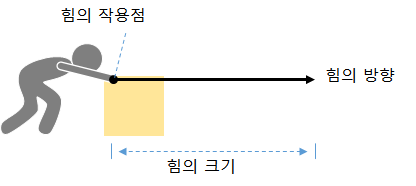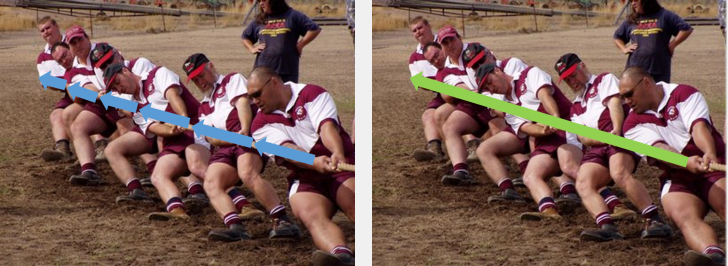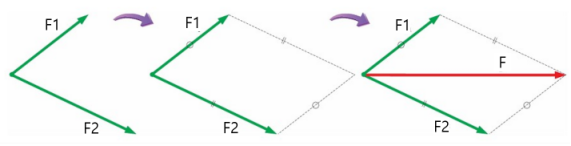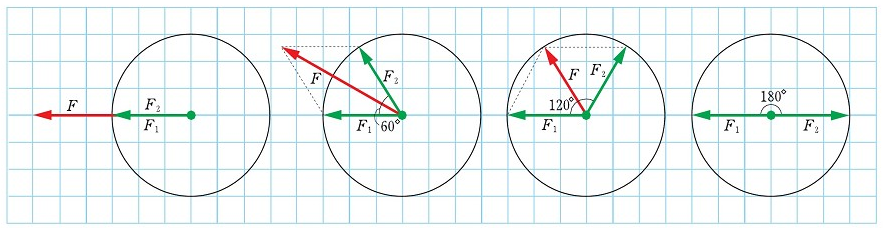## Three elements of force

When a force acts on an object, the object’s motion varies depending on the Three elements of force. That is the magnitude, direction, and point of action. Therefore, when displaying a force, you must indicate the three elements of force.When you slide a book on the desk with your finger and move it, the book moves in the finger’s direction when the finger is pushed at the middle point. If the finger is pushed at the end of the book, the book is pushed and rotated. In other words, depending on the point of action of the object, the object can only perform the translational motion, or it can also perform the rotational motion.

## The net force

When more than one force acts on an object at the same time, the single force that produces the same effect is called the net force. And the process of finding the result is called force synthesis.Together, the power of the tug-of-war can be represented by a single big arrow.

## Getting the result of the force (diagonal method)

When two forces act on an object at the same time, the sum of the two forces can be easily obtained by using the arrows. After representing the two forces with an arrow, draw two straight lines parallel to the arrow to draw a parallelogram. At this time, the diagonal arrow becomes the sum of the two forces.The magnitude of the net force between the two forces is smaller as the angle increases.
When the angle between the two forces is 0˚, it is the case that the two forces act in the same direction, so the magnitude of the net force is maximum.If the angle between the two forces is 120˚ and the two forces’ magnitudes are the same, the magnitude of the net force is equal to one force’s magnitude before addition. When the angle between the two forces is 180˚, the net force’s magnitude is the minimum because the two forces act in opposite directions.